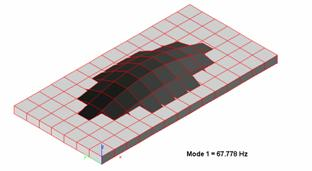“Let our rigorous testing and reviews be your guidelines to A/V equipment – not marketing slogans”

# Physics Tutorial 2: The Physics of Hearing - page 2

By Mike Duda

Pitch and Timbre
The frequency of a sound wave is the primary factor in determining the pitch of a sound, the quality that lets us classify the sound as "high" or "low". The higher the frequency of the sound within the audible range, the higher the pitch that a listener will perceive.

Pressure amplitude does play a role in determining pitch. When a listener compares two sinusoidal waves with the same frequency but different pressure amplitudes, the one with the greater pressure amplitude is usually perceived as louder but also as slightly lower in pitch .

Musical sounds have wave functions that are more complicated than a simple sine function; musical sounds consist of a fundamental frequency and harmonics. Harmonics are frequencies higher than the fundamental frequency. Two tones produced by different instruments might have the same fundamental frequency (and thus the same pitch) but sound different because of the presence of different amounts of various harmonics. The difference between the two sounds is called timber (tone color) and is often described in subjective terms such as reedy, golden, mellow or whatever else you can contrive. The same principle applies to the human voice.Figure 2.0, Harmonic Content of Two Wind Instruments

Another factor in determining tone quality is the behavior at the beginning (attack) and end (decay) of a tone. A piano tone begins with a thump and then dies away gradually.

Noise is a combination of all frequencies, not just frequencies that are integer multiples of a fundamental frequency. One example is the sound of the wind.

Sound Intensity
Sound waves, like all other traveling waves, transfer energy from one region of space to another. Intensity of a wave is the time average rate at which energy is transported by the wave, per unit area, across a surface perpendicular to the direction of propagation. In other words, intensity is the average power per unit area.

Since sound is a wave, characterized by amplitude and frequency, the mathematical formula for sound intensity includes the product of the sound wave frequency and amplitude. Lower frequencies produce smaller sound intensities. This is the reason a low-frequency woofer has to vibrate with a much larger amplitude than a high-frequency tweeter to produce the same intensity.

Lets put this into a perspective. The average total sound power emitted by a person speaking in a conversational tone is about 10 -5 Watts , while a shout corresponds to about 3 x 10 -2 Watts . If all the residents in New York City were to talk at the same time, the total sound power would be about 100 Watts, equivalent to the electric power required for a medium-sized light bulb.

If a source of sound can be considered as a point, the intensity at a distance r from the source is inversely proportional to r 2 . This "inverse-square" relationship also holds for various other energy-flow situations with a point source, such as light emitted by a point source.

The inverse-square relationship between sound intensity and distance from the source does not apply indoors because sound energy can also reach a listener by reflection from the walls and ceilings.

Because the ear is sensitive over such a broad range of intensities, a logarithmic intensity scale is usually used. The sound intensity level ß of a sound wave is defined by the equation

ß = (10 dB) log I/I o

For this equation, I o is a reference intensity, chosen to be 10^-12 W/m^2 , approximately the threshold of human hearing at 1 kHz.

If the intensity of a sound wave equals I o , its sound intensity level is 0 dB. On the opposite end of the scale, the threshold of pain is about 120 dB (1 W/m^2 ).

Since the human ear is not equally sensitive to all frequencies in the audible range, some sound-level meters weight the various frequencies unequally. One such scheme leads to the so-called dBA scale; this scale de-emphasizes the low and very high frequencies, where the ear is less sensitive than at midrange frequencies.

Summary

Tool Three: Sound is a Longitudinal Wave

1. Any mechanical wave is a disturbance that travels through some material or substance, such as air. This substance is called a medium for the wave.
2. Sound waves may also be described in terms of variation of pressure at various points in space.
3. The human ear operates by sensing pressure variations above and below atmospheric pressure.
4. The human ear is sensitive to sound waves in the frequency range from about 20 to 20,000 Hz, which is called the audible range.

Tool Four: Sound Perception

1. The physical characteristics of a sound wave are directly related to the perception of that sound by the listener.
2. An important factor that contributes to perception is that the ear is not equally sensitive to all frequencies in the audible range.
3. The higher the frequency of the sound within the audible range, the higher the pitch that a listener will perceive
4. Musical sounds consist of a fundamental frequency and harmonics.
5. Sound waves, like all other traveling waves, transfer energy from one region of space to another.
6. The sound intensity level ß of a sound wave is defined by the equation

ß = (10 dB) log I/I o